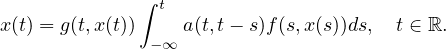Electron. J. Differential Equations, Vol. 2019 (2019), No. 79, pp. 1-11.

Existence of positive solutions for a nonlinear quadratic integral equation Chu-Hang Wang, Hui-Sheng Ding, Gaston M. N'Guerekata

Abstract:
In this article, we study the existence of positive solutions for the nonlinear quadratic integral equationBy using fixed point theory on cones, we prove the existence and uniqueness of bounded and continuous solution with positive infimum. An example illustrates the abstract result.

Submitted April 15, 2018. Published June 10, 2019.
Math Subject Classifications: 45G10, 34K37.
Key Words: Quadratic integral equation; positive solution; cone.

Show me the PDF file (323 KB), TEX file for this article.Chu-Hang Wang College of Mathematics and Information Science Jiangxi Normal University Nanchang, Jiangxi 330022, China email: 2227016700@qq.com Hui-Sheng Ding College of Mathematics and Information Science Jiangxi Normal University Nanchang, Jiangxi 330022, China email: dinghs@mail.ustc.edu.cn Gaston M. N'Guerekata Department of Mathematics Morgan State University 1700 E. Cold Spring Lane Baltimore, MD 21251, USA email: Gaston.N'Guerekata@morgan.edu